Win up to 100% scholarship on Aakash BYJU'S JEE/NEET courses with ABNAT Win up to 100% scholarship on Aakash BYJU'S JEE/NEET courses with ABNAT

# Rotational Motion

## Physics of Rotational Motion

The laws and equations that govern nature and natural phenomena are described by physics. One prime focus of physics is the study of motion. We have dealt in detail about translational motion (objects that move along a straight or curved line) in the previous chapters, and now we will expand our view towards other types of motions as well.

We see rotational motion in almost everything around us. Every machine, all celestial bodies, most of the fun games in amusement parks and if you are a FIFA fan, and when you watch David Beckham’s familiar shot, the ball is actually executing rotational motion.

Objects turn about an axis. All the particles and the mass center do not undergo identical motions. All the particles of the body undergo identical motion. By definition, it becomes essential for us to explore how the different particles of a rigid body move when the body rotates.

## Rotational Kinematics

In rotational kinematics, we will investigate the relation between kinematical parameters of rotation. We shall now revisit angular equivalents of the linear quantities: position, displacement, velocity and acceleration, which we have already dealt in a circular motion.

 Linear Kinematic Parameters Angular Kinematic Parameters Position s Angular position θ Displacement $$\begin{array}{l}\Delta s={{s}_{1}}-{{s}_{2}}\end{array}$$ Angular displacement $$\begin{array}{l}\Delta \theta ={{\theta }_{1}}-{{\theta }_{2}}\end{array}$$ Average velocity $$\begin{array}{l}{{v}_{avg}}=~\frac{\Delta s}{\Delta t}\end{array}$$ Average angular velocity $$\begin{array}{l}{{\omega }_{avg}}=~\frac{\Delta \theta }{\Delta t}\end{array}$$ Instantaneous velocity $$\begin{array}{l}{{v}_{ins}}\,\underset{\triangle t\to 0}{\mathop{\lim }}\,\frac{\Delta s}{\Delta t}=\frac{ds}{dt}\end{array}$$ Instantaneous angular velocity $$\begin{array}{l}\underset{\Delta t\to 0}{\mathop{{{\omega }_{ins}}\lim }}\,\frac{\Delta \theta }{\Delta t}=\frac{d\theta }{dt}\end{array}$$ Average acceleration $$\begin{array}{l}{{a}_{avg}}=~\frac{\Delta v}{\Delta t}\end{array}$$ Average angular acceleration $$\begin{array}{l}{{\alpha }_{avg}}=~\frac{\Delta s}{\Delta t}\end{array}$$ Instantaneous acceleration $$\begin{array}{l}{{a}_{ins}}\,\underset{\Delta t\to 0}{\mathop{\lim }}\,\frac{\Delta v}{\Delta t}=\frac{dv}{dt}\end{array}$$ Instantaneous angular acceleration $$\begin{array}{l}{{\alpha }_{ins}}\,\underset{\Delta t\to 0}{\mathop{\lim }}\,\frac{\Delta \omega }{\Delta t}=\frac{d\omega }{dt}\end{array}$$

A case of constant angular acceleration is of great importance, and a parallel set of equations holds for this case just as in constant linear acceleration.

 Linear Equations of Motion Angular Equations of Motion $$\begin{array}{l}v = v_{o} + at\end{array}$$ $$\begin{array}{l}\omega ={{\omega }_{0}}+\alpha t\end{array}$$ $$\begin{array}{l}x-{{x}_{0}}=~{{v}_{0}}+\frac{1}{2}a{{t}^{2}}\end{array}$$ $$\begin{array}{l}\theta -{{\theta }_{0}}=~{{\omega }_{0}}+\frac{1}{2}\alpha {{t}^{2}}\end{array}$$ $$\begin{array}{l}{{v}^{2}}=v_{0}^{2}+2a\left( x-{{x}_{0}} \right)\end{array}$$ $$\begin{array}{l}{{\omega }^{2}}=\omega _{0}^{2}+2\alpha \left( \theta -{{\theta }_{0}} \right)\end{array}$$

## Axis of Rotation

A rigid body of an arbitrary shape in rotation about a fixed axis (axis that does not move) called axis of rotation or rotation axis is shown in the below figure.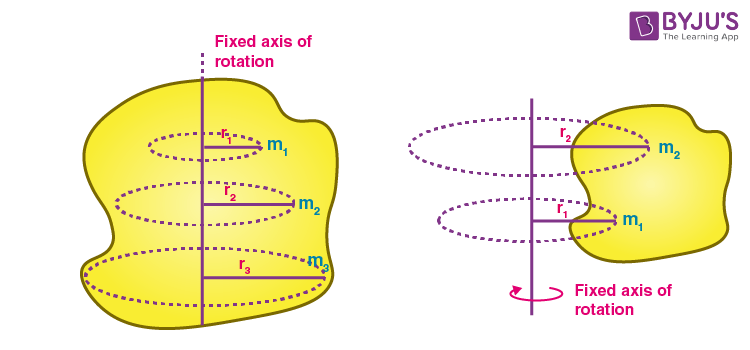### Types of Motion involving Rotation

1. Rotation about a fixed axis (Pure rotation)
2. Rotation about an axis of rotation (Combined translational and rotational motion)
3. Rotation about an axis in the rotation (rotating axis – out of the scope of JEE)

#### Rotation About a Fixed Axis

Rotation of a ceiling fan, opening and closing of the door, rotation of our planet, rotation of hour and minute hands in analogue clocks are a few examples of this type.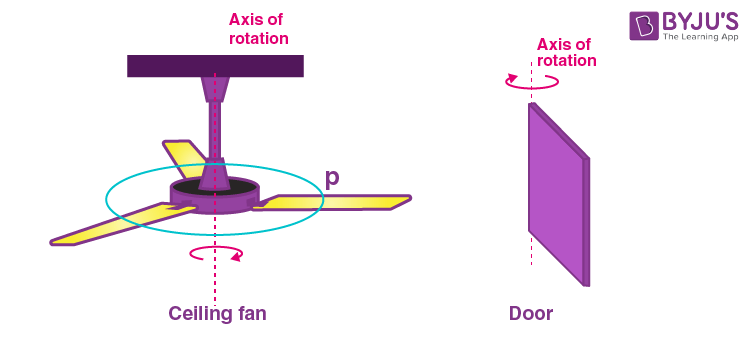#### Rotation about an axis of rotation

Rolling is an example of this category. Arguably, the most important application of rotational physics is in the rolling of wheels and wheel-like objects as our world now is filled with automobiles and other rolling vehicles.

The Rolling Motion of a body is a combination of both translational and rotational motion of a round-shaped body placed on a surface. When a body is set in a rolling motion, every particle of the body has two velocities – one due to its rotational motion, and the other due to its translational motion (of the centre of mass), and the resulting effect is the vector sum of both velocities at all particles.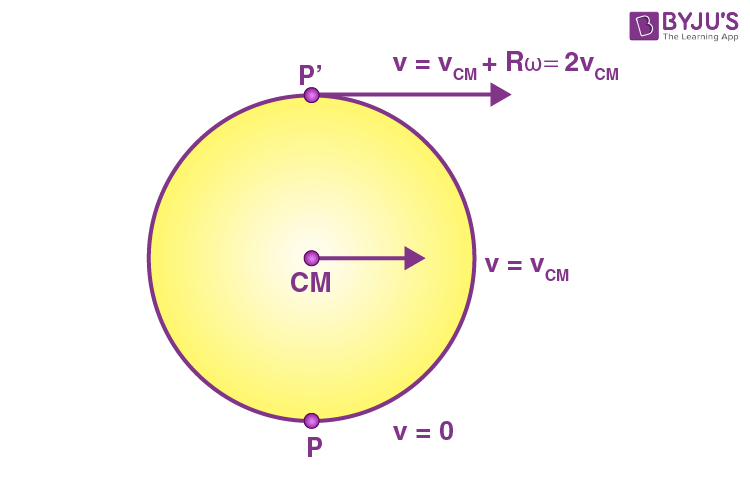There is a wheel that rotates with an angular acceleration which is given by α = 4at3 — 3bt2, where t is the time and a and b are constants. If the wheel has an initial angular speed  ω0, what are the equations for (a) angular speed (b) angular displacement.

1.

$$\begin{array}{l}\alpha = \frac{d\omega}{dt} \Rightarrow d\omega = \alpha dt\end{array}$$

$$\begin{array}{l}\Rightarrow \int_{\omega_{o}}^{\omega} = \int_{o}^{t} \alpha dt = \int_{o}^{t} (4at^{3}- 3bt^{2})dt\end{array}$$

$$\begin{array}{l}\Rightarrow \omega = \omega _{o} + at^{4} – bt^{3}\end{array}$$

2. Further,

$$\begin{array}{l}\omega = \frac{d\Theta }{dt} \Rightarrow d\Theta = \omega dt\end{array}$$

$$\begin{array}{l}\Rightarrow \int_{o}^{\Theta } = \int_{o}^{t} \omega dt = \int_{o}^{t} (\omega _{o}+at^{4}- bt^{3})dt\end{array}$$

$$\begin{array}{l}\Rightarrow \Theta = \omega _{o} + t + \frac{at^{5}}{5} – \frac{bt^{4}}{4}\end{array}$$

### Instantaneous Axis of Rotation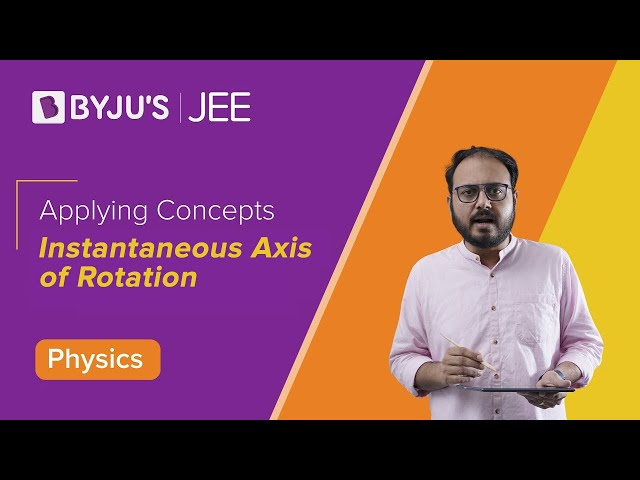### Kinetic Energy of Rotation

The rapidly rotating blades of a table saw machine and the blades of a fan certainly have kinetic energy due to rotation. If we apply the familiar equation to the saw machine as a whole, it would give us kinetic energy of its centre of mass only, which is zero.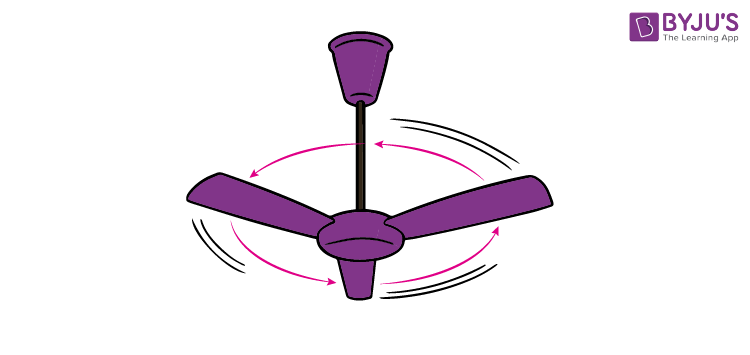The right approach:

We shall treat the saw machine or any rotating rigid body as a collection of particles at different speeds. We shall sum up all the kinetic energy of the particles to find the rotational kinetic energy of the whole body.

If m1, m2,…. mn are the masses of the constituent particles moving on circular paths with radii r1, r2,…. ro with velocities v1, v2,…. vo, then the kinetic energy of the body is given by;

$$\begin{array}{l}KE = \sum_{1}^{n}\frac{1}{2}m_{i}v_{i}^{2} = \sum_{1}^{n}\frac{1}{2}m_{i}r_{i}^{2}\omega ^{2} = \frac{1}{2}\omega ^{2} \sum_{1}^{n}m_{i}r_{i}^{2} = \frac{1}{2}I\omega ^{2}\end{array}$$

The term

$$\begin{array}{l}\sum_{1}^{n}m_{i}r_{i}^{2}\end{array}$$
is called rotational inertia or moment of inertia of the system of particles. For a continuous distribution of mass
$$\begin{array}{l}I = \int_{Body} r^{2}dm\end{array}$$
where dm is the mass of a particle at a distance r from the axis of rotation.

## What Is Torque

Torque is a rotational analogue of force and expresses the tendency of a force applied to an object that causes the object to rotate about a given point.

If you want to open a door, you will apply a force on the doorknob which is located as far as possible from the hinges of the door. If you try to apply the force nearer to the hinge line than the knob, or at any other angle other than 90ᴼ to the plane of the door, you must apply greater force than the former to rotate the door.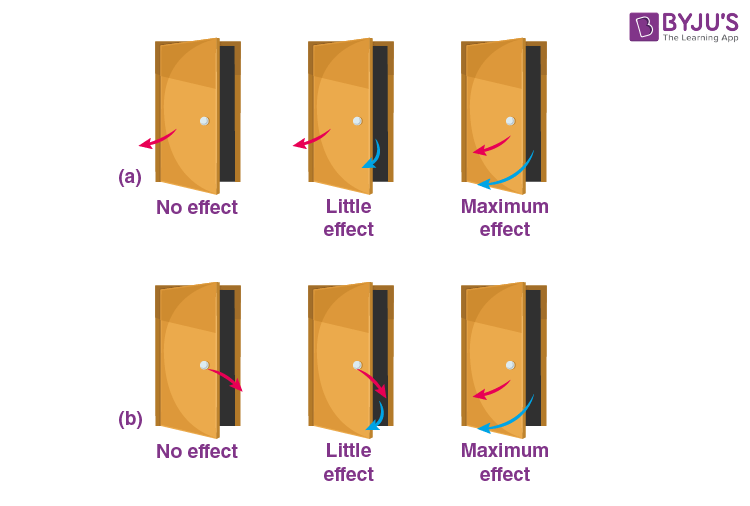To determine how the applied force results in a rotation of the body about an axis, we resolve the Force (F) into two components. The tangential component (Fsinθ) is perpendicular to r and it does cause rotation whereas the radial component (Fcosθ) does not cause rotation because it acts along the line that intersects with the axis or pivot point.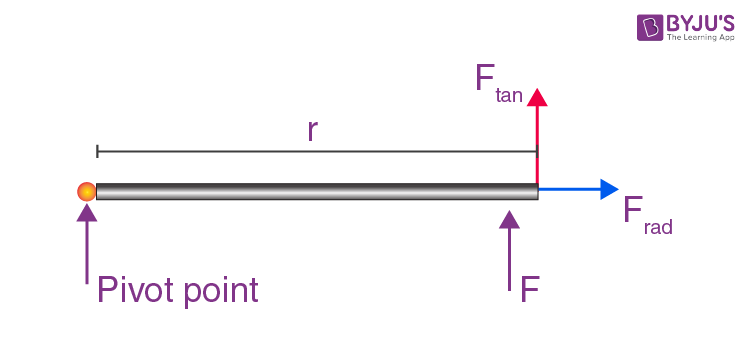The ability to rotate the body depends on the magnitude of the tangential component and also on how far from the axis the force (r – moment of an arm) is applied. Therefore, mathematically it can be represented as

$$\begin{array}{l}\vec{\tau }=\vec{r}\times \vec{F}\end{array}$$

SI unit of torque is Nm.

$$\begin{array}{l}\text{To find the direction of}\ \vec{\tau },\end{array}$$
we use right hand thumb rule sweeping the fingers from
$$\begin{array}{l}\vec{\tau }\ \text{(the first vector in the product) into}\ \vec{F}\ \text{(the second vector in the product),}\end{array}$$

$$\begin{array}{l}\text{the outstretched thumb will give the direction of}\ \vec{\tau }.\end{array}$$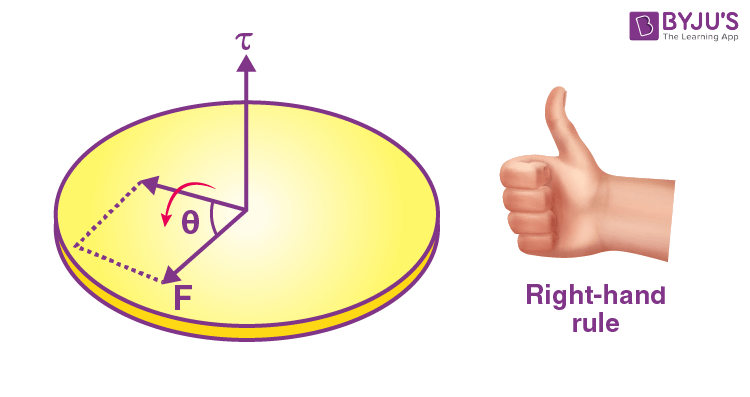### Stability of the Object

• Greater the rotational inertia of the object, an object is more stable because it is difficult to move.
• The stability of an object depends on the torques produced by its weight. Larger the torques produced if the mass is from COM, therefore more force is required to alter the stability.

Example: If you consider disk and bike wheel of the same mass, a bike wheel is more stable.

• When the object’s rotation is greater it is more stable.

### Newton’s Second Law of Rotation

$$\begin{array}{l}\text{If the net torque acting on a body about any inertial axis is}\ \vec{\tau }\end{array}$$
and the moment of inertia about that axis is I, then the angular acceleration of the body is given by the relation:

$$\begin{array}{l}\overrightarrow{~\tau }=I\overrightarrow{\alpha ~}\end{array}$$

### Rotational Equilibrium

The centre of mass of a body remains in equilibrium if the total external force acting on the body is zero. This follows from the equation F = Ma.

Similarly, a body remains in rotational equilibrium if the total external torque acting on the body is zero. This follows from the equation τ = Iα. Therefore, a body in rotational equilibrium must either be in rest or rotation with constant angular velocity.

Thus, if a body remains at rest in an inertial frame, the total external force acting on the body should be zero in any direction and the total external torque should be zero about any line.

Under the action of several coplanar forces, the net torque is zero for rotational equilibrium.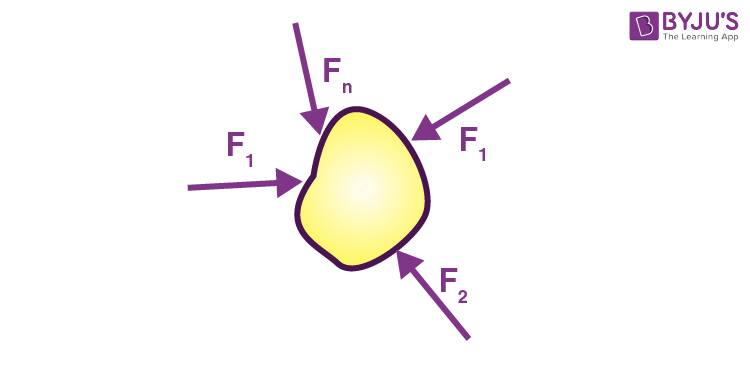Note: If the net force on the body is zero, then the net torque may or may not be zero.

Ex. Determine the point of application of third force for which the body is in equilibrium when forces of 20N & 30N are acting on the rod as shown in the figure.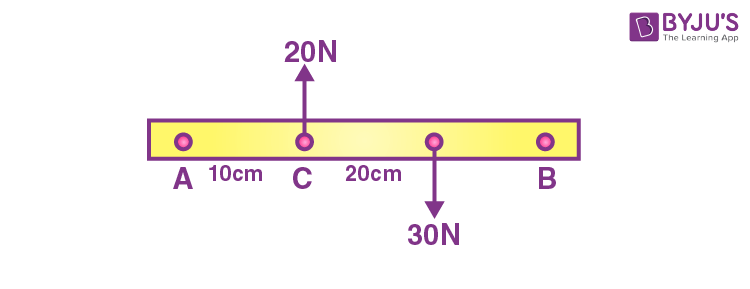Solution:

Let the magnitude of the third force is F, is applied in upward direction then the body is in equilibrium when

$$\begin{array}{l}(i)\ \vec{F}_{net}=0\end{array}$$
(Translational Equilibrium)

⇒ 20 + F = 30 ⇒ F = 10N

So, the body is in translational equilibrium when 10N force acts on it in the upward direction.

(ii) Let us assume that this 10N force acts.

Then keep the body in rotational equilibrium.

So, torque about C = 0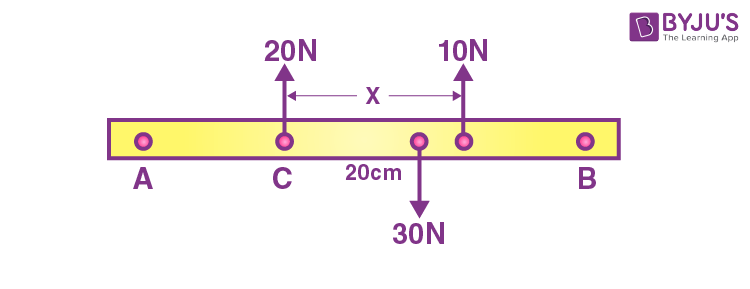i.e τC = 0

⇒ 30 × 20 = 10x

x = 60cm

So, 10N force is applied at 70cm from point A to keep the body in equilibrium.

Ex: A stationary uniform rod of mass ‘m’, length ‘l’ leans against a smooth vertical wall making an angle q with a rough horizontal floor. Find the normal force and frictional force that is exerted by the floor on the rod.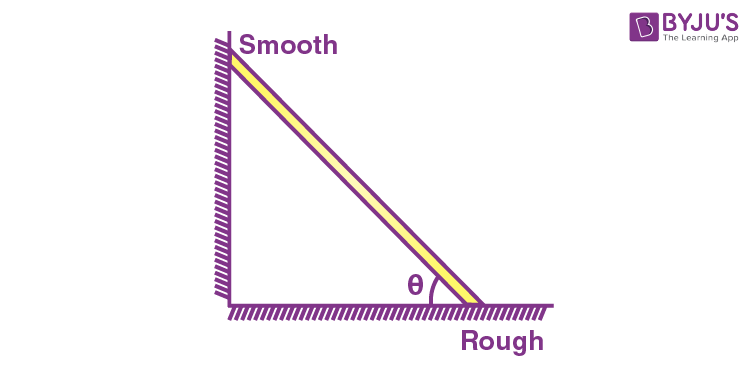Solution:

As the rod is stationary, the linear acceleration and angular acceleration of the rod are 0.

i.e. acm = 0; ɑ = 0

N2 = f

N1 = mg [∵ acm = 0]

The torque about any point of the rod should also be zero.

∴ ɑ = 0

τA = 0 ⇒ mg cos θ (l / 2) + fl sin θ = N1 cos θ. l

N1 cos θ = sin θ f + (mg cos θ) / 2

f = [mg cos θ] / [2 sin θ] = mg cot θ / 2

Ex: The ladder shown in the figure has negligible mass and rests on a frictionless floor. The crossbar connects the two legs of the ladder in the middle. The angle between the two legs is 60o. The fat person sitting on the ladder has a mass of 80 kg. Find the contact force exerted by the floor on each leg and the tension in the crossbar.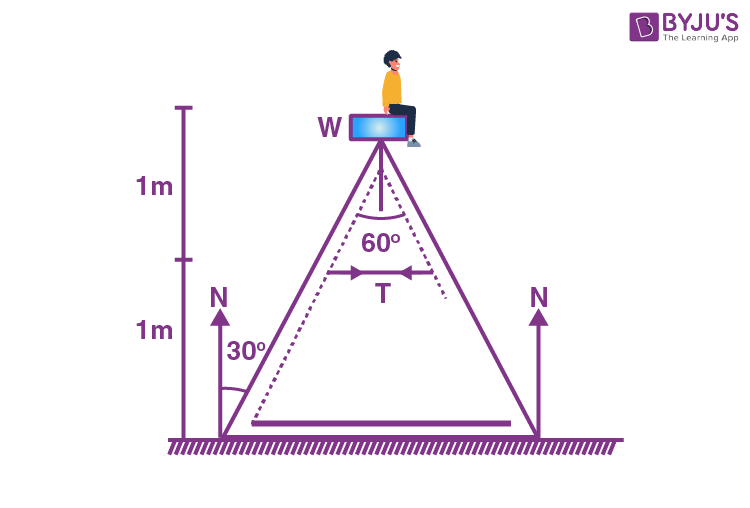Solution:

The forces acting on different parts are shown in the figure. Consider the vertical equilibrium of “the ladder plus the person” system. The forces acting on this system are its weight (80 kg) g and the contact force N + N = 2N due to the floor. Thus, 2N = (80 kg) g or N = (40 kg) (9.8 m/s2) = 392 N

Next, consider the equilibrium of the left leg of the ladder. Taking torques of the forces acting on it about the upper end,

N (2m) tan 30o = T (1m) or

T = N (2/√3) = [(392) N] * (2/√3) = 450N

Ex. The pulley shown in the figure has a moment of inertia l about the axis and radius R. Find the acceleration of the two blocks. Assume that the string is light and does not slip on the pulley.

Solution: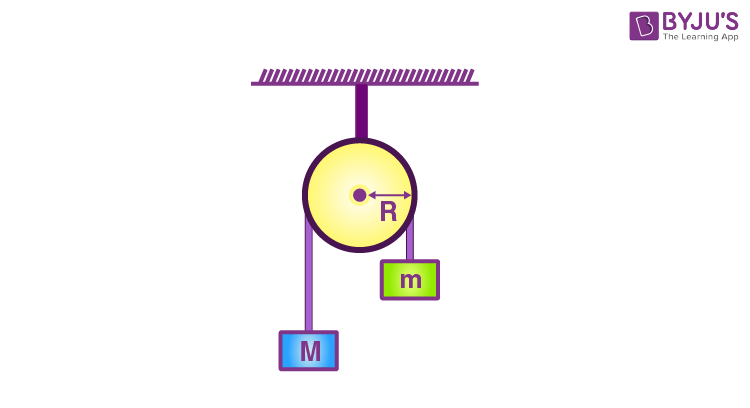Suppose the tension in the left string is T1 and that in the right string is T2. Suppose the block of mass M goes down with an acceleration a and the other block moves up with the same acceleration. This is also the tangential acceleration of the rim of the wheel as the string does not slip over the rim.

The angular acceleration of the wheel ɑ = a / R.

The equations of motion for the mass M, the mass m and the pulley are as follows;

Mg – T1 = Ma —- (i)

T2 – mg = ma —- (ii)

T1R – T2R = Iɑ = Ia / R —- (iii)

Substituting for T1 and T2 from equations (i) and (ii) in equation (iii)

[M (g – a)] – m (g + a)] R = Ia / R

Solving we get, a = [(M – m)gR2] / [I + (M + m)R2]

Angular momentum of a particle describing circular motion:

$$\begin{array}{l}\vec{L}=\vec{r}\times\vec{p}\end{array}$$
; as linear momentum
$$\begin{array}{l}\vec{p}\end{array}$$
is along with the tangent, hence
$$\begin{array}{l}\vec{r}\times\vec{p}=rpn\end{array}$$
, where n is the unit vector perpendicular to the plane of the circle.

$$\begin{array}{l}\Rightarrow \vec{|L|}=mvr=m\omega r^{2},\text{where}\ \vec{|p|}=mv=m\omega r\end{array}$$

Angular momentum of a rigid in a fixed axis rotation

In a fixed axis rotation, all the constituent particles describe circular motion. Hence, the angular momentum of the particles about corresponding centres are

L1 = m1𝛚r12, L2 = m2𝛚r22

and similarly, Ln = mn𝛚rn2 where 𝛚 is the angular speed of the body.

Since the angular momentum of all the particles have the same direction, therefore angular momentum of the whole body is given by

L = L1 + L2 +…… + Ln

= (m1r12 + m2r22 +….. + mn𝛚rn2) 𝛚

⇒ L = I𝛚

## Angular MomentumThe concept of linear momentum and conservation of linear momentum are extremely powerful tools to predict the collision of two objects without any other details of the collision. Thus, the angular counterpart, angular momentum plays a crucial role in orbital mechanics.

Angular momentum of a particle about a given point is given by,

$$\begin{array}{l}\vec{l}=\vec{r}\times \vec{p}=m\left( \vec{r}\times \vec{v} \right)\end{array}$$

The direction of angular momentum is also given by the right-hand rule. (refer torque)

Newton’s law in the angular form:

The vector sum of all the torques acting on a particle is equal to the time rate of change of the angular momentum of that particle.

$$\begin{array}{l}{{\overrightarrow{~\tau }}_{net}}=~\frac{d\vec{l}}{dt}\end{array}$$

#### Rotational Motion-Conservation of Angular Momentum### Conservation of Angular Momentum

By the definition of torque,

$$\begin{array}{l}{{\overrightarrow{~\tau }}_{net}}=~\frac{d\vec{l}}{dt},if{{\overrightarrow{~\tau }}_{net}}=0,~then~\frac{d\vec{l}}{dt}=0,\vec{l}=constant\end{array}$$

When the resultant torque acting on a system is zero, then the total vector angular momentum of the system remains constant. This is called the principle of conservation of angular momentum.

Examples of conservation of angular momentum

A planet revolves around the sun in an elliptical orbit when it goes near the sun, its speed increases and when it goes farther from the sun, its speed decreases. It happens because angular momentum is conserved, and hence time rate of the area swept by the planet is constant. A planet revolves around the sun in orbit in a fixed plane only. This is due to the conservation of angular momentum.A uniform rod of mass m and length ‘l’ can rotate freely on a smooth hor pine about a vertical axis hinged at point H. A point mass having the same mass ‘m’ coming with an initial speed u perpendicular to the rod strikes the rod in-elastically at its free end. Find out the angular velocity of the rod just after collision.Solution

Angular momentum is conserved about H because no external force is present in horizontal plane which is producing torque about H.

mul = (ml2/3 + ml2

⇒ ω = 3u/4l

Angular Impulse (Moment of Impulse)

Angular impulse of a torque in a given time is equal to the change in angular momentum. If the angular momentum of a body is changed by a torque τ in time dt then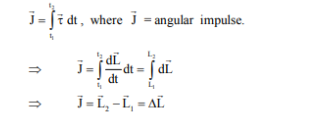### Combined Translational and Rotational Motion

Rolling motion is one such example.

Rolling motion is one such example.

To understand the concept of combined translational and rotational motion, we consider a uniform disc rolling on a horizontal surface. The velocity of its centre of mass is Vcom, and its angular speed is shown in the figure.Let us take point A on the disc and concentrate on its motion.

Path of point A with respect to the ground will be a cycloid, as shown in the figure.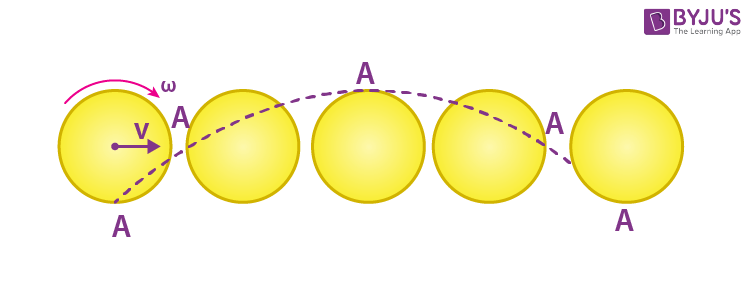Motion of point A with respect to centre of mass is pure rotational while centre of mass itself is moving in a straight line. So, for the analysis of rolling motion, we deal with translational motion separately and rotational motion separately and then we combine the result to analyse the overall motion.

The velocity of any point A on the rigid body can be obtained as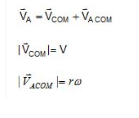in the direction perpendicular to the line OA.

Thus, the velocity of point A is the vector sum of Vcom, and Vp.com shown in figureJEE FOCUS

1. Velocity of any point of the rigid body in combined R + T motion is the vector sum of v(velocity of the centre of mass) and rω.

2, In combined rotational and translational motion, angular velocity of any point of a rigid body with respect to other point in the rigid body is always the same.

3. Distance moved by the centre of mass of the rigid body in one full rotation is 2πR.

4. The speed of a point on the circumference of the body at any instant t is 2Rωsinθ.

Angular momentum of a rigid body in combined rotation and translation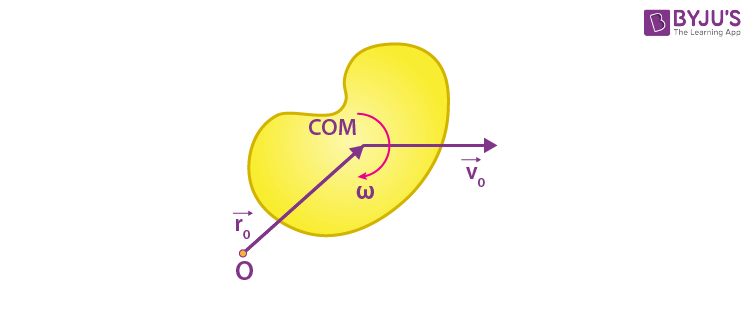Let O be a fixed point in an inertial frame of reference. Angular momentum of the body about O is.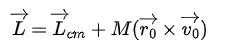The first term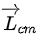represents the angular momentum of the body as seen from the centre of mass frame.

The second term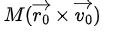equals the angular momentum of centre of mass about point O.

Example. A circular disc of mass m and radius R is set into motion on a horizontal floor with a linear speed v in the forward direction and an angular speed ω = v/R in the clockwise direction, as shown in the figure. Find the magnitude of the total angular momentum of the disc about the bottommost point O of the disc.

Solution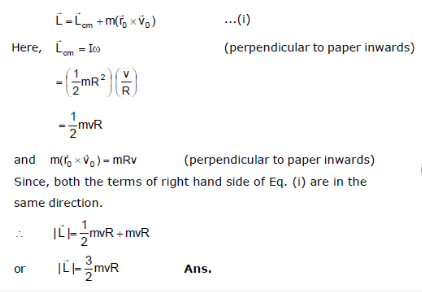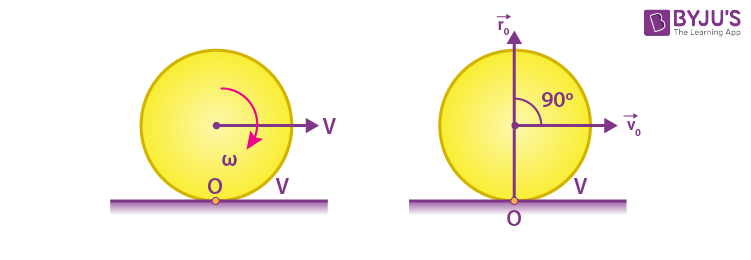Kinetic Energy of a Rolling Body

If a body of mass M is rolling on a plane such that the velocity of its centre of mass is V and its angular speed is w, its kinetic energy is given by

KE= (1/2)Mv2 + (1/2)Iω2
I is moment of inertia of body about axis passing through centre of mass.
In case of rolling without slipping,

KE= (1/2)Mω2R2 + (1/2)Iω2 [v = ωR]

= (1/2) [ MR2 + I]ω2 = (1/2)I0ω2

Ic is moment of inertia of the body about the axis passing through point of contact.

Uniform Pure Rolling
Pare rolling means no relative motion (or no slipping at point of contact between two bodies.)

For example, consider a disc of radius R moving with linear velocity v and angular velocity ω on a horizontal ground. The dis is said to be moving without slipping if velocities of points P and Q (shown in
figure b) are equal, i.e.,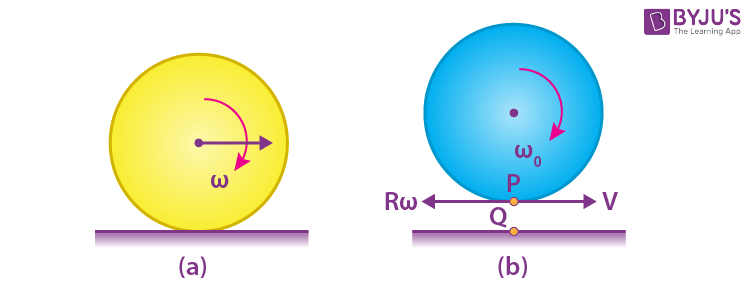vP= vQ

v – Rω = 0

v = Rω

If vp > vQ or v > Rω, the motion is said to be forward slipping and if vp < vQ < Rω, the motion is said to be backward slipping.

Now, suppose an external force is applied to the rigid body, the motion will no longer remain uniform.
The condition of pure rolling on a stationary ground is,

a=Rα

is the condition of pure rolling on the stationary ground. Sometimes it is simply said rolling.

Thus, v = Rω and a = Rα

Pure Rolling on an Inclined Plan

A rigid body of radius R and mass m is released at rest from height h on the incline whose inclination with horizontal is q and assume that friction is sufficient for pure rolling, then.

v = Rω and a = Rα

From figuremgsinθ – f = ma ——(1) [ Fnet = ma]

f.R = cm R2.(a/R) —–(2) [Fnet = Iα]

from equation (1) and (2) we get

a = gsinθ/(1+k)

Binary Systems in Nature
In a binary system, two bodies revolve about their common centre of mass due to their mutual attractive forces, i.e., no external force acts on them.

Let there be two celestial bodies of mass m1 and m2 revolving about their common centre of mass.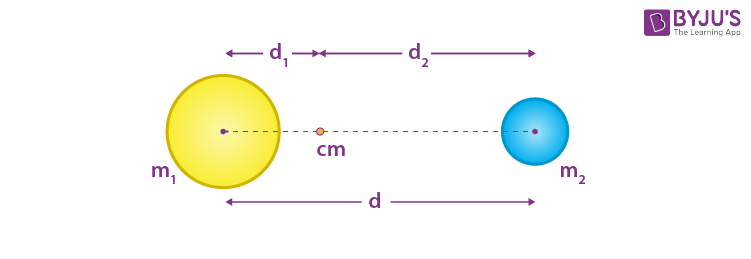By definition of centre of mass

Xcm = (m1x1 + m2x2)/(m1 +m2)

Taking centre of mass1 , as origin,

d1 = m2/(m1 +m2)d

d2=d—d1, where d1, d2 and d are as shown in the figure.

Gravitational force provides the required centripetal force, hence,

Gm1m2/d2 = m1v12/d1

Gm2/d2 = v12/d1 = (2πd1/T)(1/d1) [ since 2πd1/v1 = T]

where T is the time period of one revolution,

= 4π2d1/T2 = 4π2/T2 (m2/(m1 + m2))d

m1 + m2 = (4π2/T2G)d3

If one of the masses is known, the other mass can be calculated knowing T and d.
Typical examples of such systems are earth-moon system, binary stars.

Example. A solid sphere of radius r is gently placed on rough horizontal ground with an initial angular speed ω0 and no linear velocity. If the coefficient of friction is μ, find the linear velocity v and angular velocity w at the end of slipping.Solution

m be the mass of the sphere.

Since it is a case of backward slipping, the force of friction is in forward direction. Limiting friction will act in this case.

Net torque on the sphere about the bottommost point is zero. Therefore, angular momentum of the sphere will remain conserved about the bottommost point.

Li = Lr

0 = Iω + mrv

(2/5)mr2ω0 = (2/5)mr2ω + mr (ωr)

ω = (2/7)ωand v = rω = (2/7)ω0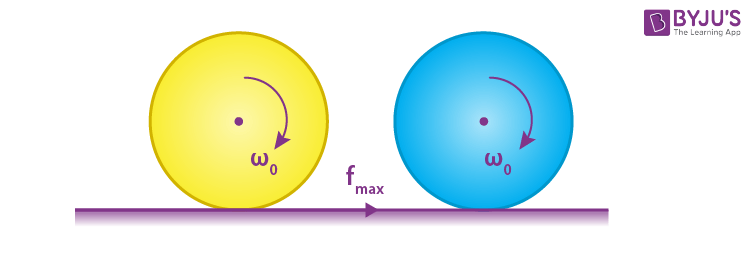Example

A sphere of radius r kept on a rough inclined plane is in equilibrium by a string wrapped over it. If the angle of inclination is θ the tension in the string will be equal to

(A)mgsinθ

(B) 2mg/5

(C) mgsinθ/2

(D) None of these

Since f & N pass through the point P, their torque about P will be zero.
For the equilibrium of the sphere, the torques of tension T and weight mg about P will be zero.= mgsinθ x r— T(2r)=0

=> 2Tr=mgr sinθ

T = mgsinθ/2

Hence (C) is correct

### Video Lesson on Work Done in Rotation### Waves and Rolling Motion – Important Questions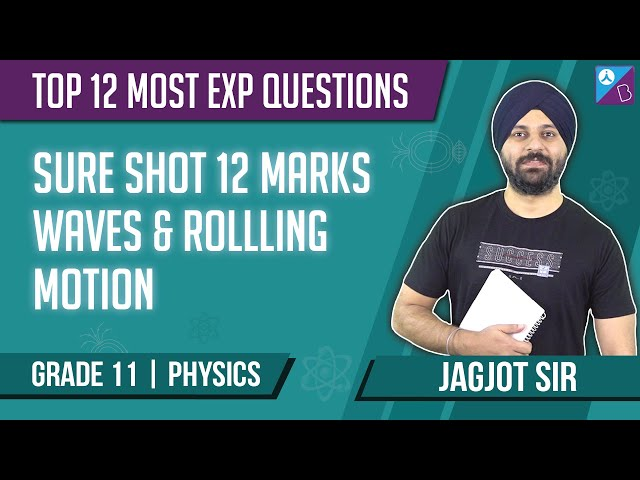## Rotational Motion – Important Topics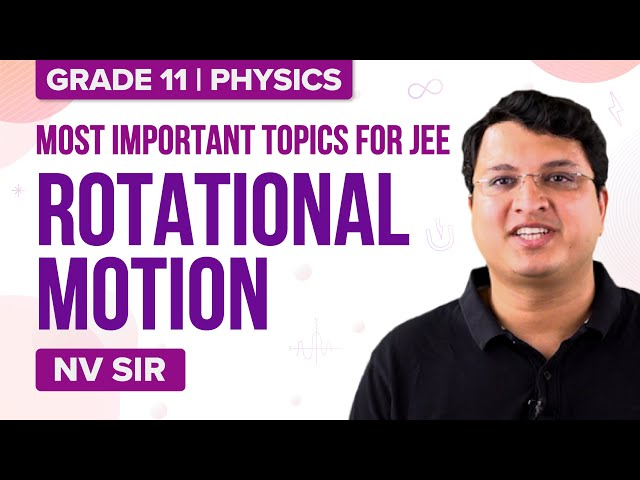## Rotational Motion – Important Questions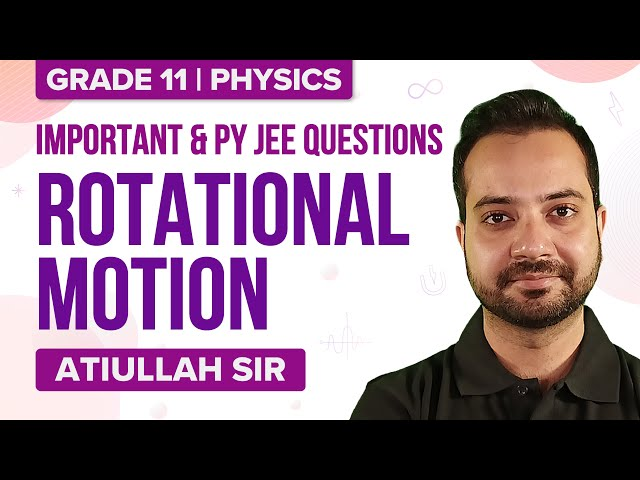## Rotational Motion for JEE Advanced – Concepts & Questions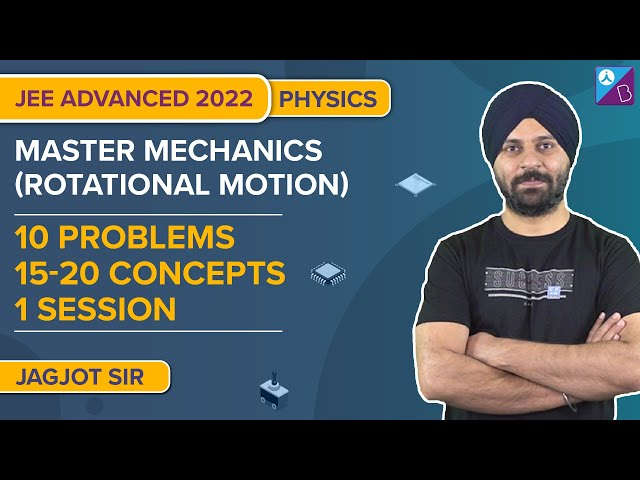## Frequently Asked Questions on Rotational Motion

Q1

### What is rotational motion? Give an example.

Rotational motion is a type of motion in which the body follows a circular path. An example is the car wheel.

Q2

### What is the reason for rotational motion?

The torque or rotational analogue force is a reason for rotational motion. When torque is applied to the system of the particle about to its axis, it gives a twist, which is the reason for rotational motion.

Q3

### Is circular motion the same as rotational motion? Explain.

Circular motion: It is a motion of the body around a fixed point. In this case, a fixed point lies outside the body. Here the centripetal force is the reason for the circular motion.
Rotational motion: In the case of rotational motion, the fixed point lies inside the body. Rotational motion is due to the torque acting on the system of the particles.

Test Your Knowledge On Rotational Motion!